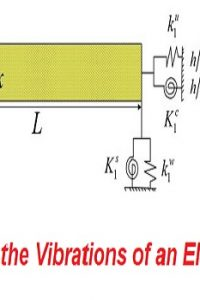اسم المؤلف
غير معروف
التاريخ
21 يوليو 2021
المشاهدات
التقييم(لا توجد تقييمات)Loading...

كتيب بعنوان
Analysis of the Vibrations of an Elastic Beam
A Fourth Order Eigenvalue/Eigenfunction Problem
cepts from the theory of elasticity it can be shown that the lateral deflection,
w( x ,t ), of a uniform, thin elastic beam of length L, set into vibration, obeys
the partial differential equation
QuickCheck Exercises
1. Find the equation determining the ay for the eigenvalues A/<; = and
the form of the eigenfunctions 4>k ( x ) for the fourth order operator (Vw ) (x) =
discussed in this section, but with the doubly damped boundary condi-
0(0) 0, g(0) = 0, 0(L) = 0, = o.
Use Newton’s method (or any other numerical equation solver you wish)
to obtain approximations, valid to four decimal places, for ay and ay as
described in f . above, taking L
d4w
on the domain S consisting of functions w( x ) with
fourth derivatives in L2|(), L\ satisfying boundary conditions
3. Let (Vw ) ( x ) dx4
Find the forms W’(°)of=the 0, eigenfunctions ^(0) = 0, w(pk ( L( x) ) and 0 the^dx2equation (L) = 0.determining
In this case the equation solved by the ay can be solved
explicitly. Find an expression for all of the solutions ay and the corresponding
eigenvalues A/c.
كلمة سر فك الضغط : books-world.net